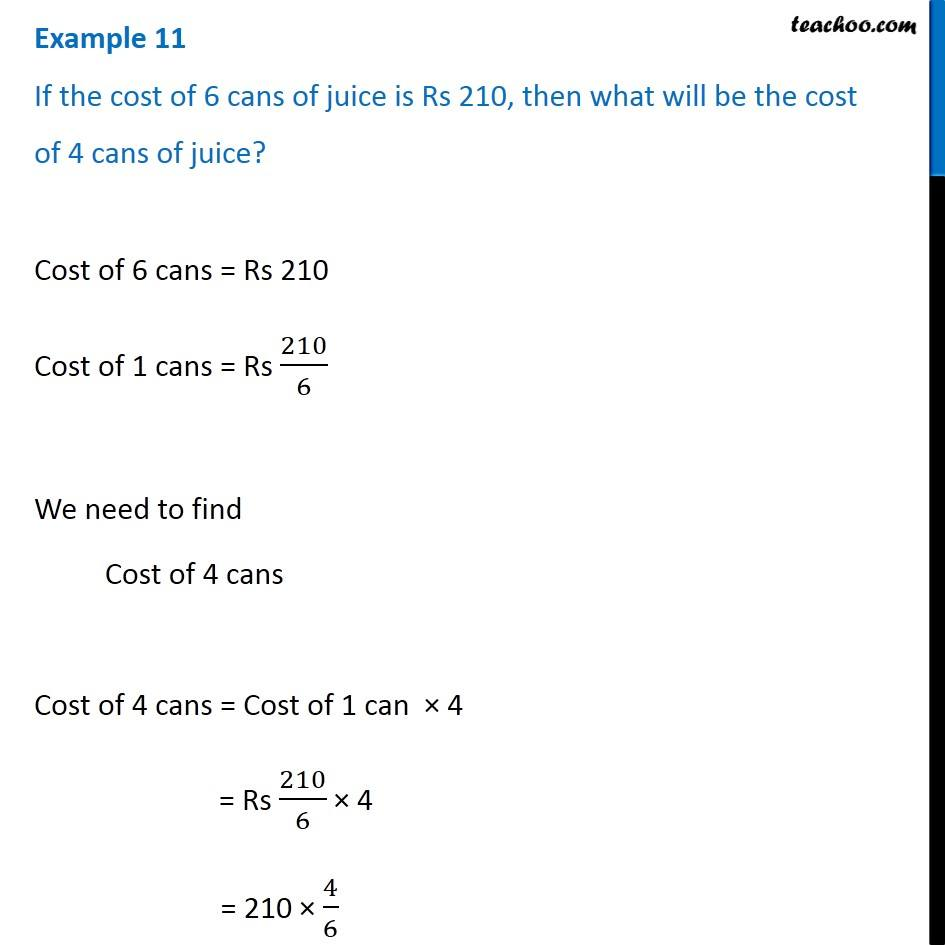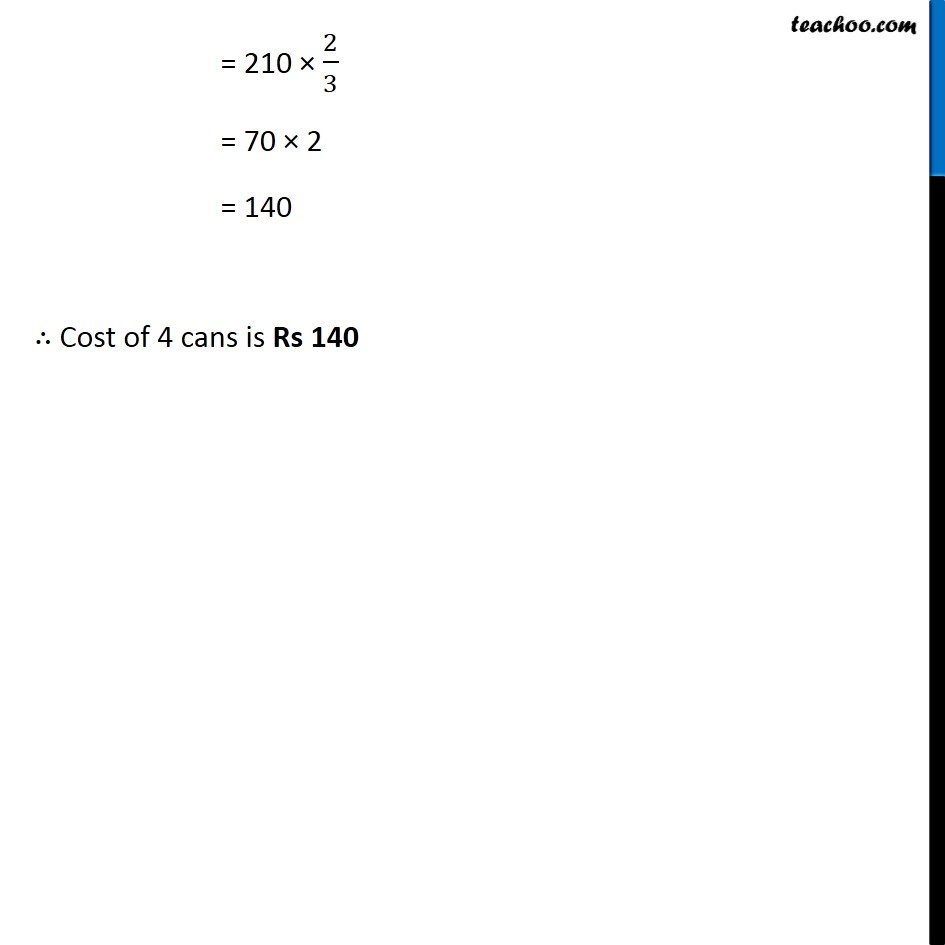Unitary Method

Chapter 12 Class 6 Ratio And Proportion
Concept wiseIntroducing your new favourite teacher - Teachoo Black, at only ₹83 per month

### Transcript

Example 11 If the cost of 6 cans of juice is Rs 210, then what will be the cost of 4 cans of juice?Cost of 6 cans = Rs 210 Cost of 1 cans = Rs 210/6 We need to find Cost of 4 cans Cost of 4 cans = Cost of 1 can × 4 = Rs 210/6 × 4 = 210 × 4/6 = 210 × 2/3 = 70 × 2 = 140 ∴ Cost of 4 cans is Rs 140 = 210 × 2/3 = 70 × 2 = 140 ∴ Cost of 4 cans is Rs 140 = 210 × 2/3 = 70 × 2 = 140 ∴ Cost of 4 cans is Rs 140